Courses
Courses for Kids
Free study material
Offline Centres
More

# NCERT Solutions for Class 7 Maths Chapter 11 - ExerciseLast updated date: 26th Nov 2023
Total views: 526.8k
Views today: 7.26k

## NCERT Solutions for Class 7 Maths Chapter 11 Perimeter and Area (EX 11.2) Exercise 11.2

Free PDF download of NCERT Solutions for Class 7 Maths Chapter 11 Exercise 11.2 (EX 11.2) and all chapter exercises at one place prepared by expert teacher as per NCERT (CBSE) books guidelines. Class 7 Maths Chapter 11 Perimeter and Area Exercise 11.2 Questions with Solutions to help you to revise complete Syllabus and Score More marks. Register and get all exercise solutions in your emails. Every NCERT Solution is provided to make the study simple and interesting on Vedantu. Subjects like Science, Maths, English will become easy to study if you have access to NCERT Solution for Class 7 Science , Maths solutions and solutions of other subjects.

## Access NCERT Solutions for Class 7 Chapter 11 – Perimeter and Area

Exercise 11.2

1. Find the area of each of the following parallelograms:

# (a)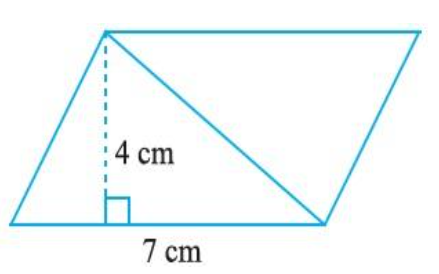Ans: Height of parallelogram is $4cm$ .

Base of the parallelogram is $7cm$ .

Therefore,

Area of the parallelogram is $base \times height$ .

The area of parallelogram is $7cm \times 4\;cm$.

So, the area of parallelogram is $28c{m^2}$ .

# (b)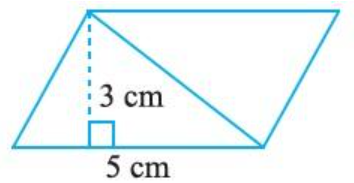Ans: Height of parallelogram is $3cm$ .

Base of the parallelogram is $5cm$ .

Therefore,

Area of the parallelogram is$base \times height$ .

Area of parallelogram is $5cm \times 3cm$

Area of the parallelogram is $15c{m^2}$ .

# (c)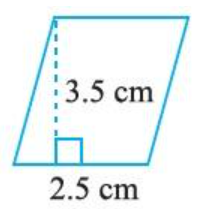Ans: Height of parallelogram is $3.5cm$ .

Base of parallelogram is $2.5cm$ .

Therefore,

Area of parallelogram is $base \times height$

Area of parallelogram is $2.5cm \times 3.5cm$

Area of parallelogram is $8.75c{m^2}$

# (d)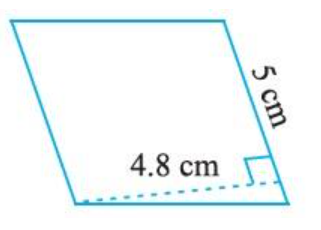Ans: Height of parallelogram is $4.8cm$.

Base of the parallelogram is $5cm$.

Therefore,

Area of the parallelogram is $base \times height$ .

Area of the parallelogram is $4.8cm \times 5cm$ .

Area of the parallelogram is $24c{m^2}$ .

# (e)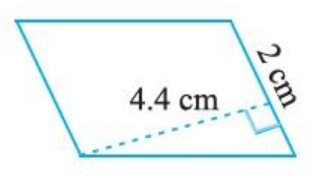Ans: Height of parallelogram is $4.4cm$ .

Base of the parallelogram is $2cm$ .

Therefore,

Area of the parallelogram is $base \times height$ .

Area of the parallelogram is $4.4cm \times 2cm$ .

Area of the parallelogram is $8.8c{m^2}$ .

2. Find the area of each of the following triangles:

# (a)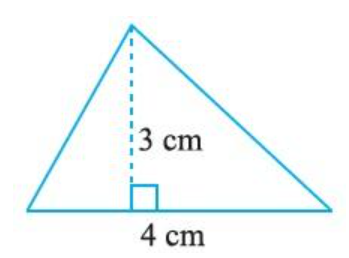Ans: Base of the triangle is $4cm$ .

Height of height is $3cm$ .

Therefore,

Area of the triangle is $\dfrac{1}{2} \times base \times height$..

Area of the triangle is  $\dfrac{1}{2} \times 4cm \times 3cm$ .

Area of the triangle is  $6c{m^2}$.

# (b)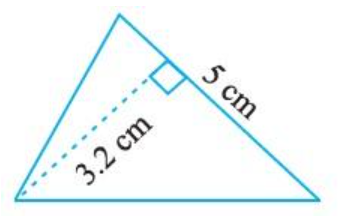Ans: Base of triangle is $5cm$ .

Height of height is $3.2cm$ .

Therefore,

Area of the triangle is  $\dfrac{1}{2} \times base \times height$.

Area of the triangle is  $\dfrac{1}{2} \times 5cm \times 3.2cm$ .

Area of the triangle is  $8c{m^2}$ .

# (c)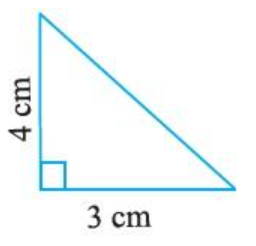Ans: Base of triangle is $4cm$ .

Height of height is $3cm$ .

Therefore,

Area of the triangle is  $\dfrac{1}{2} \times base \times height$.

Area of the triangle is  $\dfrac{1}{2} \times 4cm \times 3cm$ .

Area of the triangle is  $6c{m^2}$ .

# (d)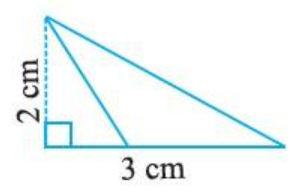Ans: Base of triangle is $3cm$ .

Height of height is $2cm$ .

Therefore,

Area of the triangle is  $\dfrac{1}{2} \times base \times height$.

Area of the triangle is  $\dfrac{1}{2} \times 3cm \times 2cm$ .

Area of the triangle is  $3c{m^2}$ .

3. Find the missing values:

 S.No. Base Height Area of the Parallelogram a. $20cm$ $246c{m^2}$ b. $15cm$ $154.5c{m^2}$ c. $8.4cm$ $48.72c{m^2}$ d. $15.6cm$ $16.38c{m^2}$

Ans (a) : Base of parallelogram is $20cm$ .

Height of the parallelogram is to be known.

Area of the parallelogram is $246c{m^2}$ .

Therefore,

$Area = base \times height$

$246c{m^2} = 20cm \times height$

$\dfrac{{246c{m^2}}}{{20cm}} = height$

$12.3cm = height$

Therefore, the height of the parallelogram is $12.3cm$ .

Ans (b) : Base of parallelogram is to be known .

Height of the parallelogram is $15cm$ .

Area of the parallelogram is $154.5c{m^2}$ .

Therefore,

$Area = base \times height$

$154.5c{m^2} = base \times 15cm$

$\dfrac{{154.5c{m^2}}}{{15cm}} = base$

$10.3cm = base$

Therefore, the base of the parallelogram is $10.3cm$ .

Ans (c) : Base of parallelogram is to be known .

Height of the parallelogram is $8.4cm$ .

Area of the parallelogram is $48.72c{m^2}$ .

Therefore,

$Area = base \times height$

$48.4c{m^2} = base \times 8.4cm$

$\dfrac{{48.4c{m^2}}}{{8.4cm}} = base$

$5.8cm = base$

Therefore, the base of the parallelogram is $5.8cm$ .

Ans (d) : Base of parallelogram is $15.6cm$ .

Height of the parallelogram is to be known.

Area of the parallelogram is $16.38c{m^2}$ .

Therefore,

$Area = base \times height$

$16.38c{m^2} = 15.6cm \times height$

$\dfrac{{16.38c{m^2}}}{{15.6cm}} = height$

$1.05cm = height$

Therefore, the height of the parallelogram is $1.05cm$ .

So, the final table is

 S.No. Base Height Area of the Parallelogram a. $20cm$ $12.3cm$ $246c{m^2}$ b. $10.3cm$ $15cm$ $154.5c{m^2}$ c. $5.8cm$ $8.4cm$ $48.72c{m^2}$ d. $15.6cm$ $1.05cm$ $16.38c{m^2}$

4. Find the missing values:

 S.No. Base Height Area of Triangle a. $15cm$ $87c{m^2}$ b. $31.4mm$ $1256m{m^2}$ c. $22cm$ $170.5c{m^2}$

Ans (a) : Base of triangle is $15cm$ .

Height of the triangle is to be known.

Area of the triangle is $87c{m^2}$ .

Therefore,

$Area = \dfrac{1}{2} \times base \times height$

$87c{m^2} = \dfrac{1}{2} \times 15cm \times height$

$\dfrac{{87c{m^2} \times 2}}{{15cm}} = height$

$11.6cm = height$

Therefore, the height of the triangle is $11.6cm$ .

Ans (b) : Base of triangle is to be known.

Height of the triangle is $31.4mm$.

Area of the triangle is $1256m{m^2}$ .

Therefore,

$Area = \dfrac{1}{2} \times base \times height$

$1256m{m^2} = \dfrac{1}{2} \times base \times 31.4mm$

$\dfrac{{1256m{m^2} \times 2}}{{31.4m}} = base$

$80mm = base$

Therefore, the base of the triangle is $80mm$ .

Ans (c) : Base of triangle is $22cm$ .

Height of the triangle is to be known.

Area of the triangle is $170.5c{m^2}$ .

Therefore,

$Area = \dfrac{1}{2} \times base \times height$

$170.5c{m^2} = \dfrac{1}{2} \times 22cm \times height$

$\dfrac{{170.5c{m^2} \times 2}}{{22cm}} = height$

$15.5cm = height$

Therefore, the height of the triangle is $15.5cm$ .

5. PQRS is a parallelogram (Fig 11.23). QM is the height from Q to SR and QN is the height from Q to PS. If SR = 12 cm and QM = 7.6 cm.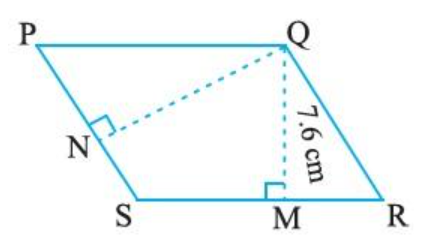Fig $11.3$

Find:

(a) The area of the parallelogram PQRS

Ans: Base of parallelogram is $12cm$ .

Height of the parallelogram is $7.6cm$ .

Therefore,

Area of parallelogram is $base \times height$

Area of the parallelogram is $SR \times QM$ .

The area of parallelogram $12cm \times 7.6cm\;$

So, the area of parallelogram is $91.2c{m^2}$.

(b) QN, if PS = 8 cm

Ans: The area of parallelogram is $91.2c{m^2}$ .

Base of the parallelogram is $8cm$ .

Height of the parallelogram is to be known.

Area of the parallelogram is $base \times height$ .

$Area = base \times height$

$Area = PS \times QN$

$91.2c{m^2} = 8cm \times QN$

$QN = \dfrac{{91.2c{m^2}}}{{8cm}}$

$QN = 11.4cm$.

6. DL and BM are the heights on sides AB and AD respectively of parallelogram ABCD (Fig 11.24). If the area of the parallelogram is $1470c{m^2}$, $AB = 35cm$cm and $AD = 49cm$, find the length of BM and DL.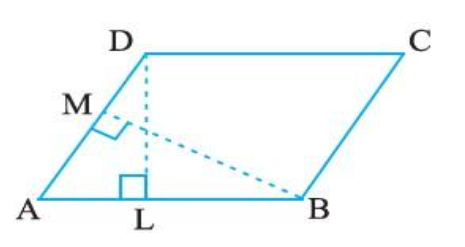Ans: Case 1 : Finding the value of DL.

Area of parallelogram is ABCD $1470c{m^2}$ .

Base of parallelogram is ,

$AB = 35cm$

Height of parallelogram is,

$DL = ?$

$Area = base \times height$

$Area = AB \times DL$

$1470c{m^2} = 35cm \times DL$

$DL = \dfrac{{1470c{m^2}}}{{35cm}}$

$DL = 42cm$

Therefore, the value of DL is $42cm$ .

Case 2: Finding the value BM.

Case 1 : Finding the value of DL.

Area of parallelogram is ABCD $1470c{m^2}$ .

Base of parallelogram is ,

$AD = 49cm$

Height of parallelogram is,

$BM = ?$

$Area = base \times height$

$Area = AD \times BM$

$1470c{m^2} = 49cm \times BM$

$BM = \dfrac{{1470c{m^2}}}{{49cm}}$

$BM = 30cm$

Therefore, the value of DL is $30cm$ .

7. $\Delta ABC$ is right angled at A (Fig 11.25). AD is perpendicular to BC. If$AB = 5cm$, $BC = 13cm$ cm and $AC = 12cm$, Find the area of $\Delta ABC$. Also find the length of AD.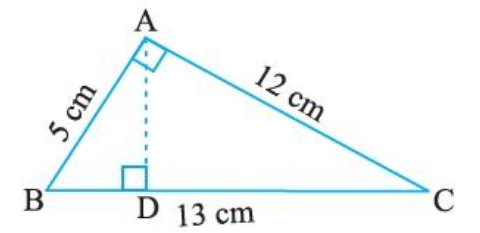Ans: Finding the area of $\Delta ABC$ .

Base of $\Delta ABC$ is $5cm$ .

Height of $\Delta ABC$ is $12cm$ .

Therefore,

$Area = \dfrac{1}{2} \times base \times height$

$Area = \dfrac{1}{2} \times AB \times AC$

$Area = \dfrac{1}{2} \times 5cm \times 12cm$

$Area = 30c{m^2}$

Therefore, the area of $\Delta ABC$ is $30c{m^2}$ .

Area of $\Delta ABC$ is $30c{m^2}$

Base of $\Delta ABC$ is $13cm$ .

Height of $\Delta ABC$ is to be known.

Therefore,

$Area = \dfrac{1}{2} \times base \times height$

$Area = \dfrac{1}{2} \times BC \times AD$

$30c{m^2} = \dfrac{1}{2} \times 13 \times AD$

$\dfrac{{30c{m^2} \times 2}}{{13cm}} = AD$

$AD = 4.6cm$

Therefore, the value of AD is $4.6cm$ .

8. $\Delta ABC$ is isosceles with AB = AC = 7.5 cm and BC = 9 cm (Fig 11.26). The height AD from A to BC, is 6 cm. Find the area of ΔABC. What will be the height from C to AB i.e., CE?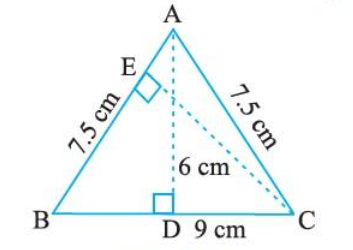Ans: Finding the area of $\Delta ABC$ .

Base of $\Delta ABC$ is $9cm$ .

Height of $\Delta ABC$ is $6cm$ .

Therefore,

$Area = \dfrac{1}{2} \times base \times height$

$Area = \dfrac{1}{2} \times AD \times BC$

$Area = \dfrac{1}{2} \times 9cm \times 6cm$

$Area = 27c{m^2}$

Therefore, the area of $\Delta ABC$ is $27c{m^2}$ .

Find the value of CE.

Area of $\Delta ABC$ is $27c{m^2}$

Base of $\Delta ABC$ is $7.5cm$ .

Height of $\Delta ABC$ is to be known.

Therefore,

$Area = \dfrac{1}{2} \times base \times height$

$Area = \dfrac{1}{2} \times BA \times CE$

$27c{m^2} = \dfrac{1}{2} \times 7.5cm \times CE$

$\dfrac{{27c{m^2} \times 2}}{{7.5cm}} = CE$

$CE = 7.2cm$

Therefore, the value of CE is $7.2cm$.

## NCERT Solutions for Class 7 Maths Chapter 11 Perimeter and Area Exercise 11.2

Opting for the NCERT solutions for Ex 11.2 Class 7 Maths is considered as the best option for the CBSE students when it comes to exam preparation. This chapter consists of many exercises. Out of which we have provided the Exercise 11.2 Class 7 Maths NCERT solutions on this page in PDF format. You can download this solution as per your convenience or you can study it directly from our website/ app online.

Vedantu in-house subject matter experts have solved the problems/ questions from the exercise with the utmost care and by following all the guidelines by CBSE. Class 7 students who are thorough with all the concepts from the Maths textbook and quite well-versed with all the problems from the exercises given in it, then any student can easily score the highest possible marks in the final exam. With the help of this Class 7 Maths Chapter 11 Exercise 11.2 solutions, students can easily understand the pattern of questions that can be asked in the exam from this chapter and also learn the marks weightage of the chapter. So that they can prepare themselves accordingly for the final exam.

Besides these NCERT solutions for Class 7 Maths Chapter 11 Exercise 11.2, there are plenty of exercises in this chapter which contain innumerable questions as well. All these questions are solved/answered by our in-house subject experts as mentioned earlier. Hence all of these are bound to be of superior quality and anyone can refer to these during the time of exam preparation. In order to score the best possible marks in the class, it is really important to understand all the concepts of the textbooks and solve the problems from the exercises given next to it.

Do not delay any more. Download the NCERT solutions for Class 7 Maths Chapter 11 Exercise 11.2 from Vedantu website now for better exam preparation. If you have the Vedantu app in your phone, you can download the same through the app as well. The best part of these solutions is these can be accessed both online and offline as well.